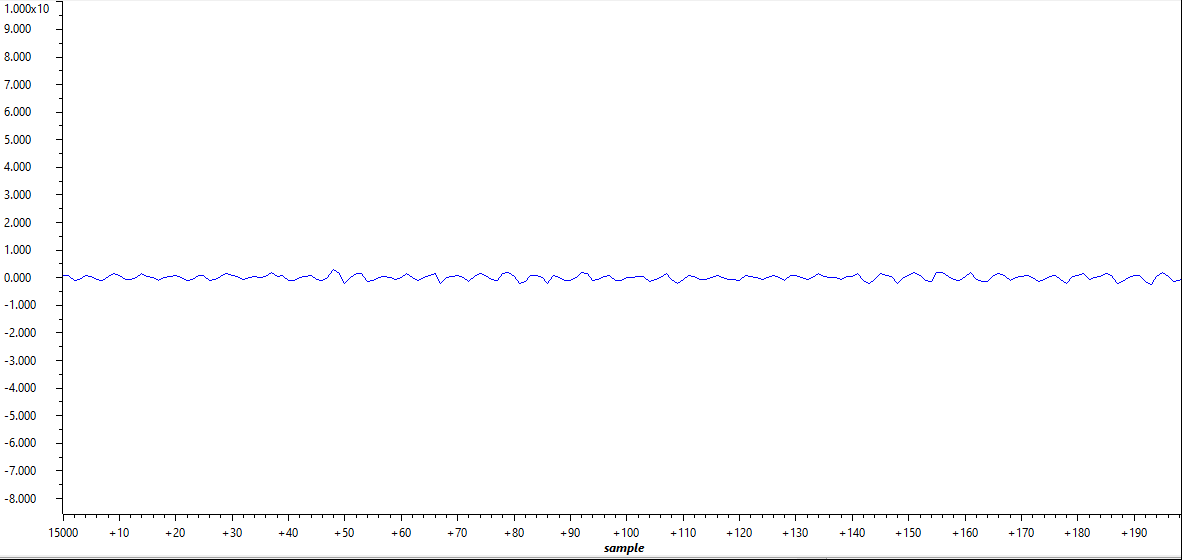2. 1Introduction
3. 2A Step-by-Step Guide to Running a Traction Inverter
4. 3A Brief Guide to Code Migration
5. 4Summary
6. 5References

### 2.4.3 Read ADC Samples in Expression and Graph Windows

ADC samples are read by the following lines 1 to 8 for 3-phase current, resolver sin/cos, and DC bus voltage. The SDK API is wrapped into Macros in one file. Couple simple "Ctrl + left click" on the variable name will help find the location where is defined. Example are given in lines 9 and 10. ADC_readResult is to read ADC result and ADC_readPPBResult is to read ADC result after Post Processing Blocks. Details on Post Processing Blocks can be find in Technical Reference Manual.

1. motor1.I_abc_A = (float32_t)IFBU_PPB;
2. motor1.I_abc_A = (float32_t)IFBV_PPB;
3. motor1.I_abc_A = (float32_t)IFBW_PPB;
4. resolver1.sin_samples = (float32_t)R_SIN1;
5. resolver1.sin_samples = (float32_t)R_SIN2;
6. resolver1.cos_samples = (float32_t)R_COS1;
7. resolver1.cos_samples = (float32_t)R_COS2;
8. motor1.dcBus_V = (float32_t)VDC_EVT;Figure 2-34 Plotted Phase A Current at No Load